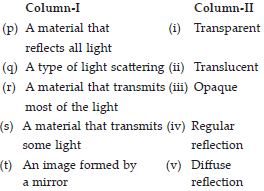# User Forum

Subject :Physics    Class : Class 7

In this section each question contains statements given in two columns which have to be matched. Statements (p, q, r, s, t) in Column-I have to be matched with statements (p, q, r, s, t) in Column-II.A p-(i), q-(ii), r-(iii), s-(iv), t-(v)
B p-(i), q-(ii), r-(iv), s-(v), t-(iii)
C p-(iii), q-(v), r-(i), s-(ii), t-(iv)
D p-(iii), q-(v), r-(i), s-(ii), t-(iv)

Options C and D are same.